Related Articles

# Tips & Tricks To Solve Ratio & Proportion – Advance Level

Ratio & Proportion is one of the most basic & easiest topics of the SSC exam. Questions from this chapter are usually asked in conjunction with the other concepts. About 3-4 questions are usually asked in preliminary examination that involves the usage of concepts of ratio & proportion.

Ratio & Proportion basics are used in almost every chapter of arithmetic portion I.e,  Profit & loss, average, partnership, percentage, etc.

### What is Ratio?

#### It tells us about the relation between the two numbers.

Example: The ratio of A to B is 3:5.
This means Value of A/ Value of B = 3/5

### What is Proportion?

#### It is the equality of two ratios. Many students often remain confused about the basic definition of Ratio & Proportion. Here we will try to clear that confusion.

A/B = 3/5 (This is the ratio of A to B)

Here, A is called the antecedent & B is called the consequent.

A/B = C/D (This is Proportion)

Proportion can also be written like,

a:b:: c:d in which Product of means= Product of extremes.

⇒ a×d = b×c

### Compounded Ratio

If two or more ratios are given, then the ratio of the product of all antecedents to the product of all consequents is called compounded ratio.

Compounded Ratio of a:b, c:d, e:f will be, ace: bdf

### Different Types of Proportion

Mean Proportion

a:M :: M:b, here M is the mean proportion of a & b.

M= √ab

Third Proportion

a:b :: b:T, here T is the third proportion of a & b.

T= b2/a

Fourth Proportion

a:b :: c:F, here F is the fourth proportion

F= (b×c)/a

Now let us try to understand some basic fundamentals of ratio that are usually used in questions asked in SSC exam through questions. We will also do some mixed problems of different topics involving the usage of ratio & proportion.

Illustration 1. If A:B = 3:4, B:C = 7:8 then find A:B:C?

Solution: B is common to both, so make B equal. Multiply 1 with 7 & 2 with 4.

⇒ A:B=21:28, B:C= 28:32

Hence, A:B:C = 21:28:32

Shortcut=  A  :   B    (B)

(A)    B   :   C

Write, the ratio in this format & then fill the blank spaces (mentioned with numbers in bracket) with the nearest number. Then, multiply vertically & you will get the answer.

Solution using shortcut=  3  :  4    (4)

(7)     7   :   8

Illustration 2. If A:B=3:4, B:C= 5:6, C:D= 7:8. Find A:B:C:D?

Solution: Now, if we approach this question using the basic method, it will take a lot of time. Use the shortcut described above to solve this problem.

A  :  B  : C  :  D

3  :  4

5  : 6

7  :  8

We have written all the given ratios in this format.

Now fill the empty spaces with the nearest number

A  :  B  : C  :  D

3  :  4   (4)   (4)

(5)   5  : 6     (6)

(7)  (7)   7  :  8

Hence the ratio =  105: 140: 168: 192

Illustration 3. If ratio is given in reciprocals, A:B:C = 1/4 : 1/7 : 1/12. Convert it

Solution. Multiply the numerator with 4×7×12, we get

A : B : C = 7× 12 : 4× 12: 4×7 = 84: 48: 28 = 21: 12: 7

Illustration 4. If 2 numbers are in the ratio of 4:7. If 9 is added to both the numbers, the ratio becomes 7:10. Find the sum of the original numbers?

Solution: When 9 is added to both the numbers, 4 becomes 7 (in antecedent) & 7 becomes 10 (in consequent). It means the addition of 9 is equivalent to 3 units.

⇒ 1 unit= 3

Now we have to find some of the original numbers, i.e (4+7)×3 = 33

Illustration 5.  If the Ratio of A:B in a mixture is 4:9 and after adding 9 litres of liquid B the ratio now becomes 1:3. Find the original quantity if liquid A in the mixture?

Solution. A:B is 4:9, after adding 9 litres of new, the ratio is 1:3.

As only B is added, the quantity of A should remain the same. Making the quantity of A equal in both the ratios by multiplying second by 4, we get 4: 12.

Now, compare both the ratios for B, 12-9 units = 9 litres

⇒ 1 unit = 3 litres. Hence, 4 units, i.e quantity of liquid A is 12 litres.

Illustration 6. The mean proportion of 9 & 25 is M. Third proportion of M & 15 is  T. Find the fourth proportion of T, 18 & 30

Solution. M = √9×25 = 15

Third proportion of M & 15 = (15×15)/ 15 = 15 = T

Fourth proportion of T, 18 & 30 = (18 × 30)/ 15 = 36

Illustration 7. There are 3 containers with equal capacity. The ratio of water to oil in the first container is 4:3, in the second container is 9:5 & in the last container is 13:8. If these containers are mixed together. Find the ratio of water to oil in the resultant mixture.

Solution. As capacity is equal, so, making the capacity equal first & then adding.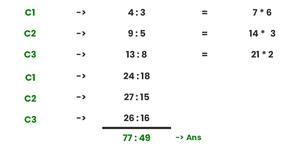Water: oil = 77:49

Illustration 8. 150 litres of a mixture contains milk & water in a ratio of 13:2. After the addition of some more milk to it, the ratio of milk to water now becomes 9:1. The quantity of milk that was added to the mixture was?

Solution. The concept to catch here is that water remains the same in both, so equate that in both ratios. We get, x = 5 units. 15 units = 150 L, so the water added is 50 litres.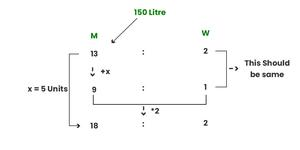Illustration 9. A container has a mixture of 2 liquids in a ratio of 7:5. When 9 litres of the mixture are drawn off & filled with B, the ratio now becomes 7: 8. Find the quantity of the initial mixture?

Solution. When we took 9 litres out of the mixture the first time, it will not bring any change in the ratio. Then 9 litres of B is added, which gives us 3 units = 9 L, i.e 1 unit = 3 Litres. Now, the total quantity of the mid mixture is, (7+5)× 3= 36 litre. So total of initial mixtures = 36+9 = 45 litres.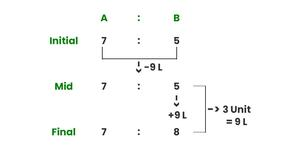Illustration 10. The annual income of Varsha & Pooja is in the ratio of 3:2, while the ratio of their expenditure is 4:3. If they save 2000 & 1000 respectively. Then Varsha’s income is?

Solution. This is a shortcut to solving questions like this. First write both the ratios, then savings. Cross multiply & take the difference as shown below. It gives us 1 unit = 2000. So, Varsha’s income is 3 × 2000 = 6000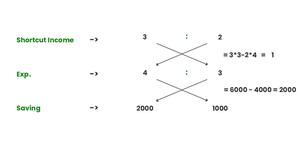Illustration 11. A man divides his property among his 3 sons in the ratio of 7: 4: 2 If the middle son gets, 18000 Rs more than the younger son. Find out how much more the elder son got than the combined money of the middle & the younger son.

Solution. Given 4x – 2x = 18000, x= 9000

Now asked, 7x – 6x= x = 9000

Illustration 12: 564 Rs was to be divided among A, B & C in the ratio of 1/3 : 1/4 : 1/5. But by mistake, it was divided into 3:4:5. The amount in excess received by C was?

Solution. The excess amount from the picture below is 235-144= 91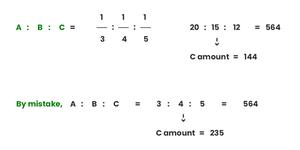Illustration 13. A man covers a long journey by train, bus & rikshaw in the ratio 4:5:2. The ratio of the fair is 2:3:1. The total expenditure was 500. Then the expenditure on the train was?

Solution. As shown in the picture below, this question is based on a compounded ratio. Expenditure on train= 160 Rs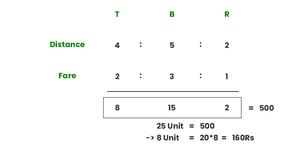Illustration 14. If we increase the price of the movie ticket in the ratio 10:11. The number of tickets sold decreases in the ratio of 10:9. Then the loss in the revenue of the theatre is?

Solution.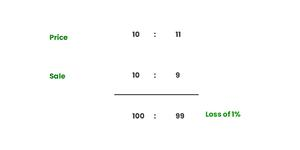Illustration 15. The ratio of the sum of salaries of Raju & Mohan to the difference in their salary is 10:1. Also, the ratio of the sum of salaries of Mohan to Isha is 7:2. If the sum of their salaries is 16400. Find the salary of Mohan?

Solution. Now, R+M+I = 16400 = 25

Mohan salary is (9 × 16400)/25 =164 * 36 = 5904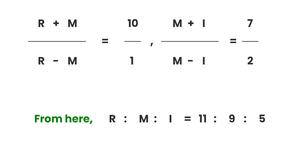Whether you're preparing for your first job interview or aiming to upskill in this ever-evolving tech landscape, GeeksforGeeks Courses are your key to success. We provide top-quality content at affordable prices, all geared towards accelerating your growth in a time-bound manner. Join the millions we've already empowered, and we're here to do the same for you. Don't miss out - check it out now!

Previous
Next